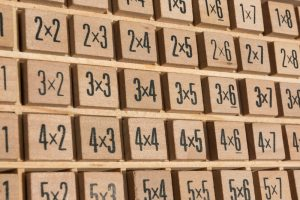Traditionally, the multiplication facts (often referred to as the times tables) are taught in numeric order, i.e. the ones facts, followed by the twos, etc. By using the suggested strategies (and building on what students already know) an alternative order of introducing the facts can be applied.

If students know (or can quickly familiarise themselves) their ones and tens facts, the teacher would then introduce the twos (doubles), fours (double, doubles) and eights (double, double, doubles). The fives facts are the tens facts halved. The threes are the twos plus the ones. The sixes are the fives plus the ones. The sevens are the fives plus the twos and finally, the nines are the tens subtract the ones.More information on this alternative  method for introducing the multiplication facts can be found by  clicking on the button below.

The following multiplication grid may be useful for students to help them keep track of which facts they are able to recall.

Recommended Posts